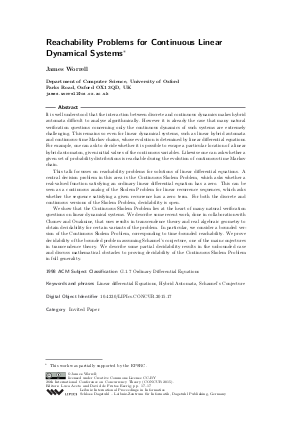Document# Reachability Problems for Continuous Linear Dynamical Systems (Invited Paper)

### Author James Worrell## File

LIPIcs.CONCUR.2015.17.pdf
• Filesize: 235 kB
• 1 pages

## Cite As

James Worrell. Reachability Problems for Continuous Linear Dynamical Systems (Invited Paper). In 26th International Conference on Concurrency Theory (CONCUR 2015). Leibniz International Proceedings in Informatics (LIPIcs), Volume 42, p. 17, Schloss Dagstuhl - Leibniz-Zentrum für Informatik (2015)
https://doi.org/10.4230/LIPIcs.CONCUR.2015.17

## Abstract

It is well understood that the interaction between discrete and continuous dynamics makes hybrid automata difficult to analyse algorithmically. However it is already the case that many natural verification questions concerning only the continuous dynamics of such systems are extremely challenging. This remains so even for linear dynamical systems, such as linear hybrid automata and continuous-time Markov chains, whose evolution is detemined by linear differential equations. For example, one can ask to decide whether it is possible to escape a particular location of a linear hybrid automaton, given initial values of the continuous variables. Likewise one can ask whether a given set of probability distributions is reachable during the evolution of continuous-time Markov chain. This talk focusses on reachability problems for solutions of linear differential equations. A central decision problem in this area is the Continuous Skolem Problem, which asks whether a real-valued function satisfying an ordinary linear differential equation has a zero. This can be seen as a continuous analog of the Skolem Problem for linear recurrence sequences, which asks whether the sequence satisfying a given recurrence has a zero term. For both the discrete and continuous versions of the Skolem Problem, decidability is open. We show that the Continuous Skolem Problem lies at the heart of many natural verification questions on linear dynamical systems. We describe some recent work, done in collaboration with Chonev and Ouaknine, that uses results in transcendence theory and real algebraic geometry to obtain decidability for certain variants of the problem. In particular, we consider a bounded version of the Continuous Skolem Problem, corresponding to time-bounded reachability. We prove decidability of the bounded problem assuming Schanuel's conjecture, one of the main conjectures in transcendence theory. We describe some partial decidability results in the unbounded case and discuss mathematical obstacles to proving decidability of the Continuous Skolem Problem in full generality.
##### Keywords
• Linear differential Equations
• Hybrid Automata
• Schanuel's Conjecture

## Metrics

• Access Statistics
• Total Accesses (updated on a weekly basis)
0